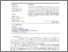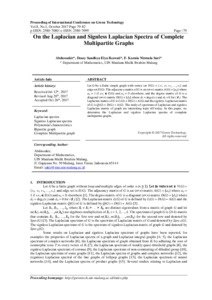# On the Laplacian and signless Laplacian spectra of complete multipartite graphs

Abdussakir, Abdussakir and Ikawati, Deasy Sandhya Elya and Sari, F. Kurnia Nirmala (2017) On the Laplacian and signless Laplacian spectra of complete multipartite graphs. Presented at The 8th International Conference on Green Technology, 8 October 2017, Malang.Preview
Text (full text)
3683.pdf - Published Version

Full text available at: http://conferences.uin-malang.ac.id/index.php/ICGT...

## Abstract

Let G be a finite simple graph with vertex set V(G) = {v1, v2, v3, …, vn} and edge set E(G). The adjacency matrix of G is an (nn)-matrix A(G) = [aij] where aij = 1 if vivj  E(G) and aij = 0 elsewhere, and the degree matrix of G is a diagonal (nn)-matrix D(G) = [dij] where dii = degG(vi) and dij = 0 for i ≠ j. The Laplacian matrix of G is L(G) = D(G) – A(G) and the signless Laplacian matrix of G is Q(G) = D(G) + A(G). The study of spectrum of Laplacian and signless Laplacian matrix of graph are interesting topic till today. In this paper, we determine the Laplacian and signless Laplacian spectra of complete multipartite graphs.

Item Type: Conference (Paper) Laplacian spectra, Signless Laplacian spectra, Polynomial characteristics, Bipartite graph, Complete multipartite graph 01 MATHEMATICAL SCIENCES > 0101 Pure Mathematics > 010104 Combinatorics and Discrete Mathematics (excl. Physical Combinatorics) Faculty of Mathematics and Sciences > Department of Mathematics Abdussakir Abdussakir 12 Jul 2018 10:35View Item